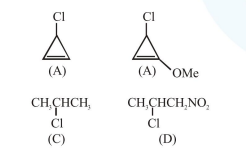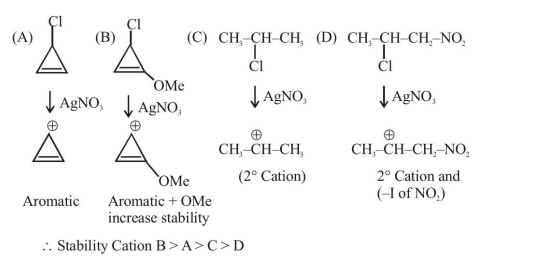# The decreasing order of reactivity of the following organic molecules

Question:

The decreasing order of reactivity of the following organic molecules towards $\mathrm{AgNO}_{3}$ solution is :1. $(\mathrm{A})>(\mathrm{B})>(\mathrm{D})>(\mathrm{C})$

2. $(\mathrm{A})>(\mathrm{B})>(\mathrm{C})>(\mathrm{D})$

3. $(\mathrm{C})>(\mathrm{D})>(\mathrm{A})>(\mathrm{B})$

4. $(\mathrm{B})>(\mathrm{A})>(\mathrm{C})>(\mathrm{D})$

Correct Option: , 4

Solution: